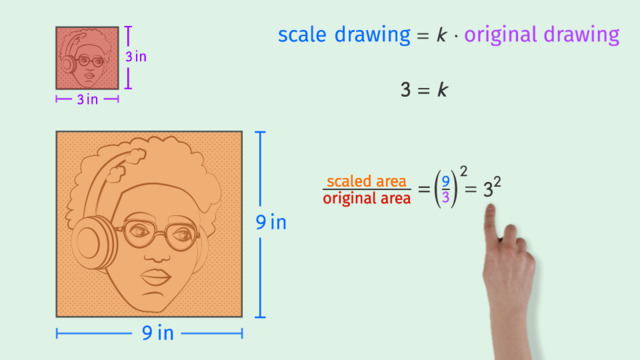# Solving Area Problems Using Scale DrawingsRating

Be the first to give a rating!

The authorsTrentkay

## Basics on the topicSolving Area Problems Using Scale Drawings

After this lesson you will be able to solve area problems related to scale drawings.

The lesson begins by finding the scale factor or constant of proportionality, k. It leads to using the scale factor to describe the relationship between the areas of drawings. It concludes with learning that the scaled area can be found by multiplying the square of k times the original area.

Learn how to solve area problems using scale drawings by helping Malik make his very first zine!

This video includes key concepts, notation, and vocabulary such as: scale factor (the constant of proportionality, k, between two similar objects); and the formula, scaled area equals k squared times original area.

Before watching this video, you should already be familiar with area of shapes, the notation for “k squared”, and the equation Quantity = Percent x Whole.

After watching this video, you will be prepared to solve more advanced word problems involving scale factors and percents.

Common Core Standard(s) in focus: 7.RP.A.2b, 7.G.A.1